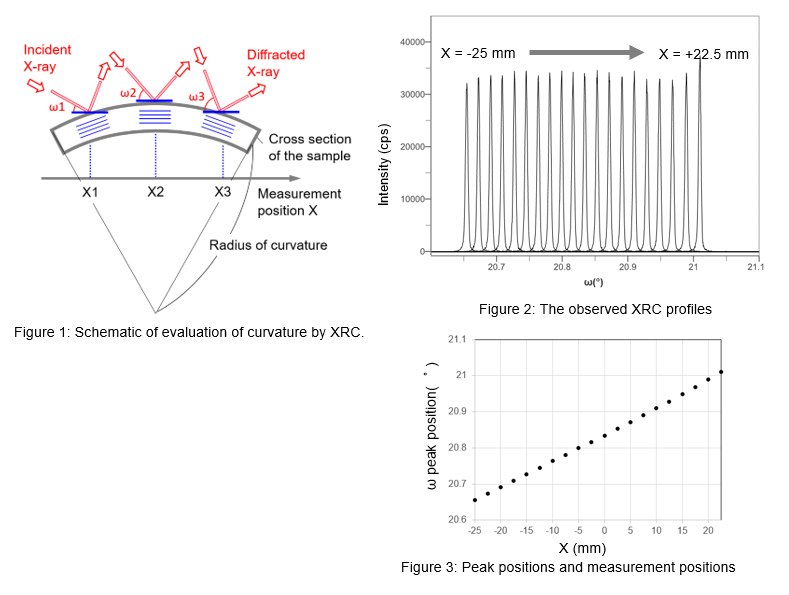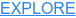# EVALUATION OF CURVATURE OF A SINGLE CRYSTAL SUBSTRATE BY ROCKING CURVE MEASUREMENT

### Introduction

Curvature of a single crystal substrate may occur during the thin film fabrication process because of differences in thermal expansion coefficients between the substrate and the film material. Since curvature can affect the subsequent manufacturing process and characteristics of products, quantitative evaluation of the degree of curvature is required. When a single crystal substrate is curved, the orientation of the lattice planes changes depending on the position on the sample (Figure 1). This change can be evaluated by an X-ray rocking curve (XRC) measurement and converted to the curvature radius by a simple equation.

### Measurements and Results

XRC measurement for sapphire 0006 reflection was performed on GaN (2 μm thick)-fabricated c-plane sapphire substrate at 2.5 mm intervals along the incident direction using a 0.1 mm x 0.5 mm X-ray beam.
Figure 2 shows XRC profiles observed at each measurement position. The peak position shifted to a higher angle as the X position is traversed. This indicates that the sample has a convex curvature, as shown in figure 1 (on the other hand, when the sample has a concave curvature, the peak position shifts to a lower angle). Figure 3 shows the relationship between the X position and the XRD peak position. The larger the curvature, the larger the slope of the graph. The radius of curvature is calculated by r = ΔX / sin(Δω). For this sample, the radius of curvature is calculated to be 7.7 m. Additionally, the constant inclination of the slope means the curvature is uniform across the sample.Recommended equipment
► Automated multipurpose X-ray diffractometer SmartLab + XY 4-inchφ attachment head

## Rigaku Recommends

#### SmartLab## MULTIPURPOSE X-RAY DIFFRACTION SYSTEM WITH BUILT-IN INTELLIGENT GUIDANCE